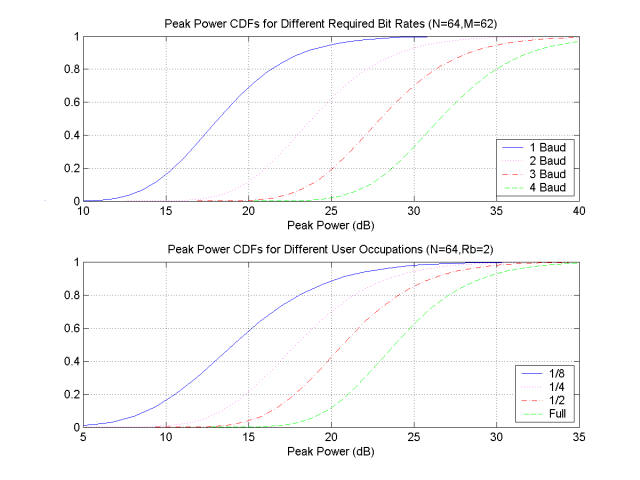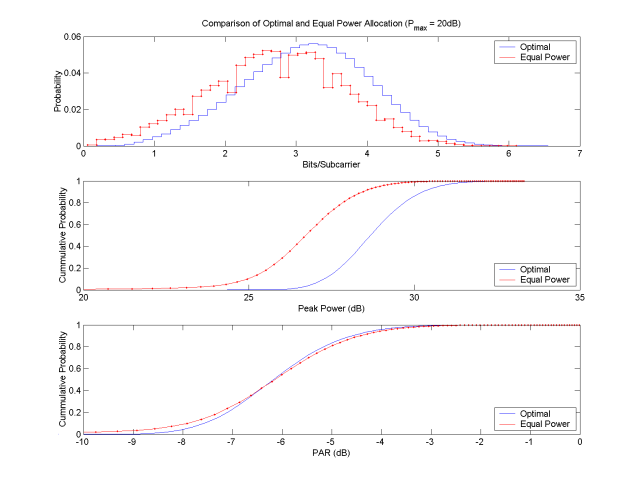# EE360 Project: Wideband Multiple Access using OFDM: Implementation and Capacity

## Project Abstract

PDF Abstract

Information theoretic results have shown that good bit, power and user-frequency allocation leads to optimal loading of frequency-selective wireless multiple access and broadcast channels. Using OFDM, several pseudo-optimal techniques have been derived that approach these optimum. For real, mobile systems, however, there is a key issue - transmitter linearity - that has a direct effect on the achievable capacity. This paper examines the relationship between the power efficiency of an optimal two user OFDM FDMA system and its capacity. An analytic expression for two-user capacity in a fading environment with a soft-limited power amplifier non-linearity is derived, and a modification to the optimal bit, power, and subcarrier algorithm is presented. A two user multiple access system [most likely assuming perfect symbol synchronization at the receiver] is simulated in a Rayleigh fading environment, and throughput reduction due to the non-linearity is shown.

## Project Update

PDF Project Update

## Project Report

PDF Project Report

## Results

Preliminary single user results shown in Figure 1 reveal an interesting characteristic of the optimal single-user power allocation. A two-user multiple access system was simulated, in which half of the 64 subbands (real transmission assumed, implying only 32 actual channels) were randomly assigned to one user, with a six-tap exponentially decaying Rayleigh distributed channel impulse response. A time-correlated Rayleigh channel was simulated in which 1024 samples were generated for each channel (and tap), with a sampled Doppler frequency of 0.0511 (which is admittedly somewhat high).

Depicted are the cumulative distribution functions of the peak power of three systems.  In the optimal scheme, the number of bits and the transmit power in each subcarrier were adjusted to minimize overall transmit power using the approach outlined in .  In the suboptimal scheme, only power was adjusted, to meet the given rate requirement.  In the third scheme, a flat, rather than fading channel is shown.    The rate for each user was specified, the right half of the figure is a 1bit/subcarrier system, and the left half a 4 bits/subcarrier system.

Figure 1.

Figure 2 depicts the dependence of the peak power distribution on required bit rate (baud, or per OFDM symbol), and channel occupation (inversely proportional to the number of users in a FDMA system).  Two interesting characteristics are noticed here.  First, the shapes of the distributions change both with increasing baud rate and increasing occupation.  Second, it is somewhat clear from the pictures that the variance of the distributions also changes.  In the top figure, this makes intuitive sense, as the input distribution should become more Gaussian as the baud rate increases.Figure 2.

The next section involves a similar simulation as above.  Instead of assuming a fixed rate constraint for the users, now a maximum power is assumed.  Figure 3 shows a comparison of optimal single user bit and power allocation versus what we call an "equal power" allocation.  "Equal power" is the equivalent of equal bit allocation above, except now, instead of fixing the number of bits per subcarrier, the amount of power per subcarrier is fixed.  It is not surprising that the optimal scheme achieves (in general) a higher bit rate.  What is surprising is that the difference is only about 0.5 bits/subcarrier.  This may be a result of several factors, including the channel model, the power constraint chosen and depicted below, or even the fact that this simulation was done assuming full channel occupation.  The last suggestion is the most relevant.  Next we will examine the situation when multiple users occupy the channel simultaneously.Figure 3.

## References

1. EE360 Class notes.